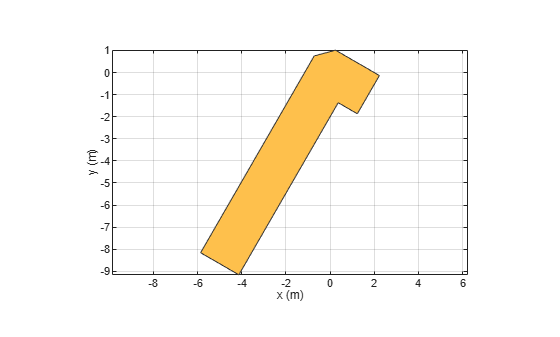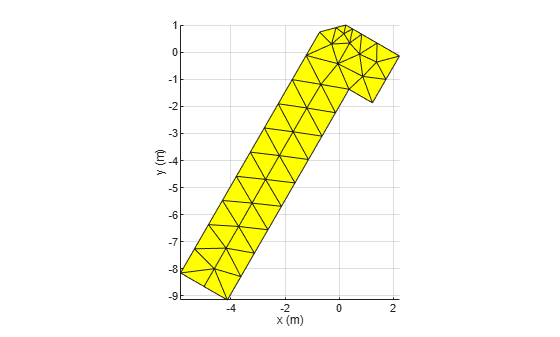# mesh

Change and view mesh properties of metal or dielectric in PCB component

## Syntax

``mesh(object)``
``mesh(shape)``
``mesh(___,Name,Value)``
``meshdata = mesh(___,Name,Value)``

## Description

````mesh(object)` plots the mesh used to analyze a PCB component.```
````mesh(shape)` plots the mesh for the shapes.```

example

````mesh(___,Name,Value)` changes and plots the mesh structure of a PCB component, using additional options specified by the name-value pairs. You can also determine the number of unknowns from the number of basis functions in the output.```
````meshdata = mesh(___,Name,Value)` returns a mesh structure that specifies the properties used to analyze the PCB component.```

## Examples

collapse all

Create a mitered bend shape of lengths of 10 m and 2 m, width of 2 m, and rotate it about the Z-axis by 60 degrees.

```bend = bendMitered(Length=[10 2],Width=[2 2],MiterDiagonal=1); bend = rotateZ(bend,60)```
```bend = bendMitered with properties: Name: 'myMiteredbend' ReferencePoint: [0 0] Length: [10 2] Width: [2 2] MiterDiagonal: 1 ```
`show(bend)`Mesh the mitered bend shape at a maximum edge length of 1 m.

`mesh(bend,MaxEdgeLength=1)`## Input Arguments

collapse all

PCB component, specified as an object handle. For complete list of PCB components and shapes, see PCB Components Catalog

Shape created using custom elements and shape objects, specified as an object handle. For a complete list of custom shapes, see Custom Geometry and PCB Fabrication.

Example: `c = bendCurved; mesh(c)`

### Name-Value Arguments

Example: ‘MaxEdgeLength’, 0.1

Specify optional comma-separated pairs of `Name,Value` pair arguments. `Name` is the argument name and `Value` is the corresponding value. `Name` must appear inside single quotes (`''`). You can specify several name and value pair arguments in any order as `Name1, Value1, ..., NameN, ValueN`.

Maximum edge length of triangles in mesh, specified as a comma-separated pair consisting of `'MaxEdgeLength'` and a scalar. All triangles in the mesh have sides less than or equal to the `'MaxEdgeLength'` value.

Data Types: `double`

Minimum edge length of triangles in mesh, specified as a comma-separated pair consisting of `'MinEdgeLength'` and a scalar. All triangles in the mesh have sides greater than or equal to the `'MinEdgeLength'`.

Note

You can use this property only with the `pcbComponent` object.

Data Types: `double`

Mesh growth rate, specified as a comma-separated pair consisting of `'GrowthRate'` and a scalar. The default value of `0.7` states that the growth rate of the mesh is 70 percent. Growth rate values lie between `0` and `1`.

Note

You can use this property only with the `pcbComponent` object.

Data Types: `double`

Customize mesh view of a PCB component, specified as a comma-separated pair consisting of `'View'` and `'all'`, `'metal'`,`'dielectric surface'`, or `'dielectric volume'`.

You choose `'dielectric surface'` to view the boundary triangle mesh of the dielectric. You choose `'dielectric volume'` to view the tetrahedral volume mesh of the dielectric.

Data Types: `char`

## Version History

Introduced in R2021b